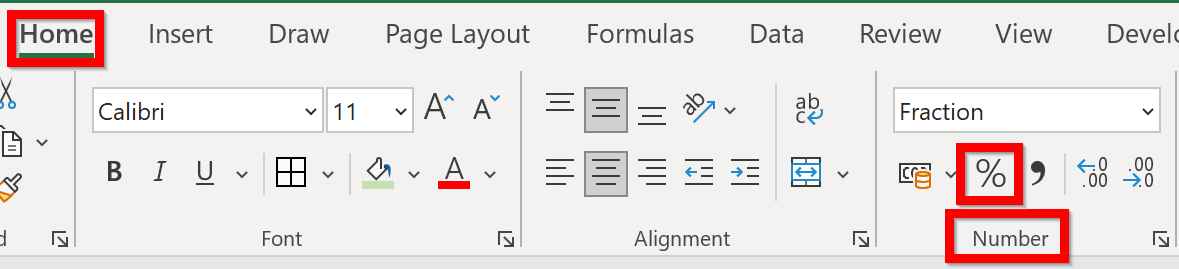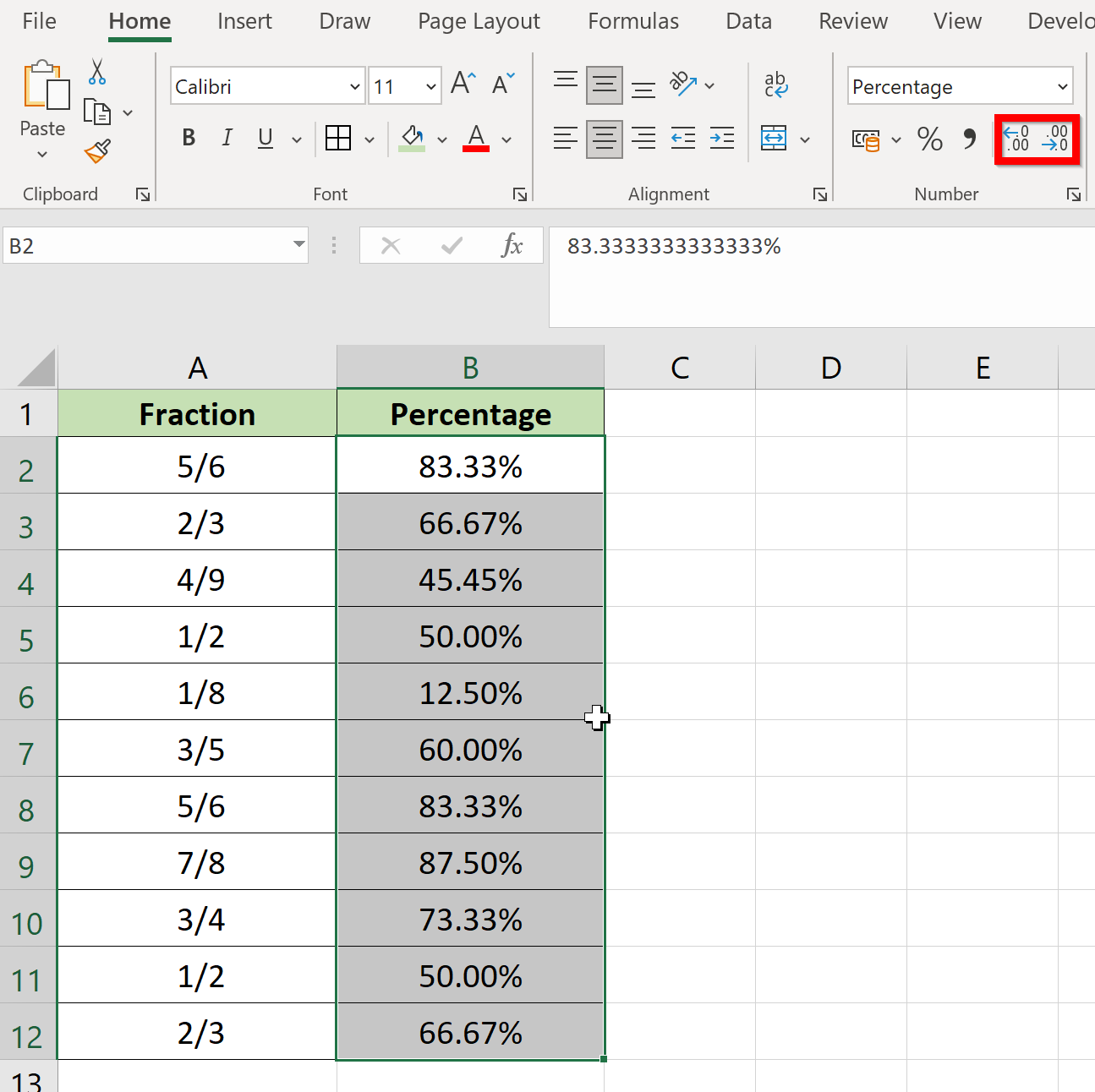# How to Format Fractions to Percentages in Excel

This guide will explain how you can use Excel to format fractions into percentages.

Excel includes built-in number format commands and custom format options that you can use to convert fractional values into a percentage format.

While decimals are often used in Excel spreadsheets, you may still encounter fractional values in a dataset. For example, when working with recipes, the instructions may require ¼ teaspoon of salt or ½ cup of cooking oil.

Fractions in Excel must use the Fraction format. If no fraction format is applied to a cell, it may be formatted as a date. For example, 1/2 will be interpreted as January 1st.

Since fractional data is simply decimal data re-formatted as a fraction, Excel gives users the option to convert between the two. Excel also allows you to convert fractions into percentages since they are both ways to express the same value.

Let’s take a look at a quick example of a situation where we might need to convert fractions into a percentage value.

You have a sheet that contains a list of discounted items. Each row includes the name of the item, its original price, and the discount rate written in fractions. For example, your sheet indicates that a specific phone case is initially worth \$15 but now offers a discount of 1/4th of the price.

When advertising discounted items, you would prefer to show the percentage value of the discount rather than the fraction. You would prefer to say that an item is 25% off rather than using the fraction 1/4.

With the built-in number format commands, you can convert your fractions into percentage data quickly. We can also use the more advanced Custom Format options to specify how many decimals to include in the final percentage.

Now that we have an idea of what tools to use to format fractions to percentages, let’s look at how these tools can be used on an actual sample spreadsheet.

## A Real Example of Formatting Fractions to Percentages in Excel

Let’s take a look at a real example of the number formatting command being used in an Excel spreadsheet.

In the table below, we have a list of fractions in cell A and a corresponding list of percentage values in column B. We’ve used a number format command to convert a copy of the values in column A into percentages.In the second example below, we’ve used custom formatting to format the fraction to a percentage value that includes up to two decimal places.You can make your own copy of the spreadsheet above using the link attached below.

If you’re ready to try out these tools to format fractions in Excel, let’s start writing it ourselves step-by-step in the next section.

## How to Format Fractions to Percentages in Excel

This section will guide you through each step needed to convert fractions to a percentage format in Excel. You’ll learn how we can use both number format commands and custom formatting options to achieve this.

Note that these steps do not affect the underlying value of the cell. Formatting in Excel only affects how users see a number in a spreadsheet.

First, follow these steps to start using the number format command:

1. Select the cell or cell range you want to convert into a percentage. In this example, we’ve selected the range B2:B12.2. Next, click on the fraction command in the Number section under the Home tab.3. Your cell range should now have the proper formatting. For example, our value of ½ in cell A5 has now been converted to the equivalent percentage value of 50%.4. You can choose to use the Increase Decimal or Decrease Decimal commands to specify how many decimals you want to show.If you want to specify the number of decimal places to include when converting to percentages, you can use the Custom Format options.

1. First, click on the More Number Formats… option in the dropdown menu seen in the Number section of the Home tab.2. Next, select the Custom option for the Category field. Under Type, you must provide a string that indicates how many decimals to include in the result. For example, the string ‘0.00%’ indicates that you would like to have two decimal places in the final result.## Frequently Asked Questions (FAQ)

1. Does this method work for mixed numbers?
Mixed numbers refer to a whole number with a fraction. For example, the value 2 ½ is a mixed number. As long as the mixed number is valid, our method will still output the correct decimal equivalent.This step-by-step guide should be all you need to format fractions into percentages in Excel. Our guide shows how easy it is to use both the custom formatting options and the number format commands in the Home tab.

Formatting fraction data is just one possible use case for Excel’s powerful formatting options. With so many other Excel functions available, you can surely find a few that you can use on your own data.

Are you interested in learning more about what Excel can do? Subscribe to our newsletter to find out about the latest Excel guides and tutorials from us.### Get emails from us about Excel.

Our goal this year is to create lots of rich, bite-sized tutorials for Excel users like you. If you liked this one, you'd love what we are working on! Readers receive ✨ early access ✨ to new content.

##### You May Also Like## How to Ignore Blanks When Calculating the Weighted Average in Excel

Learning how to ignore blanks when calculating the weighted average in Microsoft Excel is useful when you want…## How to Remove Drop-down List in Excel

This guide will explain how you can remove a drop-down list in Excel. Since drop-down lists are a…## How to Display Numbers as Fractions in Excel

This guide will discuss how to display numbers as fractions in Excel using two simple and efficient ways. …## How to Fix Formula Not Updating Automatically in Excel

This tutorial will explain a few ways to fix formulas not updating automatically in Microsoft Excel. By default,…